Search IntMath
Close

450+ Math Lessons written by Math Professors and Teachers

5 Million+ Students Helped Each Year

1200+ Articles Written by Math Educators and Enthusiasts

Simplifying and Teaching Math for Over 23 Years

# Logarithms - a visual introduction

By Murray Bourne, 10 May 2010

One of my regular correspondents, Yousuf, recently became very confused over what "log" meant. He'd missed a small, but vital piece of information − that if the base is omitted, it's assumed we are talking about log base 10.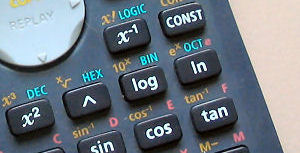Calculator showing "log" and "ln" buttons.

So "log" (as written in math text books and on calculators) means "log10" and spoken as "log to the base 10". These are known as the common logarithms.

We use "ln" in math text books and on calculators to mean "loge", which we say as "log to the base e". These are known as the natural logarithms.

Many of my students would incorrectly write the second one as "In" (as in In spring, the flowers bloom.)

But how could you blame them? In the font used on most calculators (see photo above), and in many books, it looks more like an "I" (I am hungry) than an "l" (There is a lamb).

And when we need to find the natual logarithm of the number 1, it looks like this:

ln 1

If the spacing is bad, it looks like this:

ln1

Depending on the font used, this could even be confused with this

|n| (the absolute value of n)

Wouldn't it be better for all if it was the following?

loge1

I feel "log" and "ln" is terrible notation and it is not surprising students get confused. The concept of logarithms is already somewhat foreign, so why make it even more difficult with bad notation?

## Where did logarithms come from?In the early 17th century, due to colonization by the Europeans, world trade was really taking off. There was intense interest in astronomy, since this increased the chances of a ship coming back with its bounty. Clockmakers were also in great demand. All of this required more and more sophisticated calculation.

Activities like banking and trade resulted in huge volumes of calculation, and accuracy was essential. For example, compound interest and the distances of moons, planets and stars involved a large number of multiplications and divisions. But this was very tedious and time-consuming, as well as being prone to error.

Surely there had to be a better way?

Logarithms were developed in the early 17th century by the Scotsman John Napier and the Englishman Henry Briggs (who later suggested base 10 rather than Napier's strange choice). Their ideas were refined later by Newton, Euler, John Wallis and Johann Bernoulli towards the end of the 17th century.

Napier gave us the word "logarithm", from the Greek λoγoς (logos) meaning ratio, and αριθμoς (arithmos, meaning "number"). In Napier's new system, the difference of two logarithms determines the ratio of the numbers they represent, so that an arithmetic series of logarithms corresponds to a geometric series of numbers.

## Example

To understand what the above means, let's look at a multiplication example. We are going to multiply the following (without using calculator):

69.18 × 776.25

We could start multiplying (and this would involve 20 multiplications, including carries, in 4 rows plus one row of addition, involving 8 additions plus carries), but imagine what would be involved if there were 7 or 8 digits in each number!

## Solution Involving Logarithms

We draw the curve y = 10x.

We locate 69.18 on the y-axis (point A) and move horizontally until we hit the curve y = 10x. Then we go vertically down until we hit the x-axis. The value of x we find is 1.84. (They actually would have found this value using a set of tables.)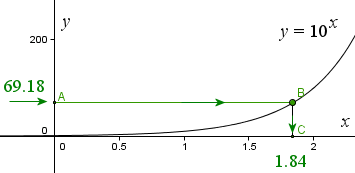We do the same thing with the other number. Start at 776.25 on the y-axis, intersect the curve at E, then project down to the x-axis, landing at 2.89.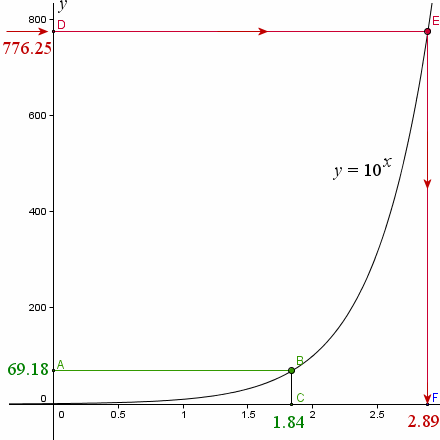Now, to calculate our multiplication problem, we add the two x-values we found:

1.84 + 2.89 = 4.73

Next, to find the final answer, we do the reverse process. We project 4.73 (point G, at bottom right) up to the curve (point H), then across to the y-axis (point J, which they'd also find using tables).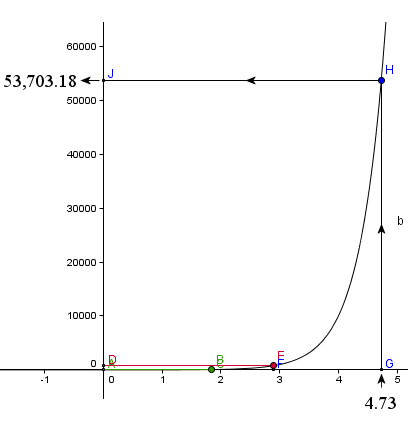We see we have landed at 53,703.18, which is our solution.

What we have done is to multiply 69.18 by 776.25 and obtained 53,703.18, with out doing any multiplication at all - just adding.

In effect, what we were doing with the graphs was actually using logarithms and we have done the following:

101.84 × 102.89 = 101.84+2.89 = 104.73

All logarithm notation does is to let us write the above statement without indices (powers). The equivalent statement using logarithms is:

log1069.18 + log10776.25

= 1.84 + 2.89

= 4.73

In the last step of the graph process above, we found the "antilogarithm" of 4.73. In effect, an antilogarithm of a number is 10 raised to the power of that number. That is,

antilog(4.73) = 104.73 = 53703.18

The various log laws are based on the fact when we multiply 2 numbers with the same base, we just add indices (like we did in the example above). See more here: Log Laws.

Logarithm notation is also a function notation, which is more convenient for calculation than if we use powers of 10.

## Division Using Logarithms

To perform difficult divisions, you would just subtract the logarithms, rather than add them. The rest of the process was the same. You could find square roots by finding 1/2 of the logarithm.

Note: The accuracy of log tables was an issue for developers. The actual answer for 69.18 × 776.25 using calculator is 53700.975. So working to 2 decimal places throughout has given us an error of around 0.004 of 1% - not much, but still significant when you are talking about distances to other stars. Since our original numbers (69.18 and 776.25) had 4 and 5 significant digits respectively, our final answer can only be accurate to 4 significant figures (the lesser of 4 and 5). So our answer using logs should be written 53700 (the first zero is significant) and this tallies with the calculator answer correct to 4 significant figures. (See Operations with Approximate Numbers on this page Approximate Numbers for more detail.)

## Log Tables

For 250 years, logarithms were the primary method for reducing complicated calculations into easier ones. A huge amount of effort was put into creating logarithm tables with as many decimal places as possible for best accuracy. These allowed people to find the logarithm of any number, and after performing the easier calculation, to do the opposite process, and find the antilogarithm.

Logarithm tables were used in schools around the world until the 1980s, when calculators took over.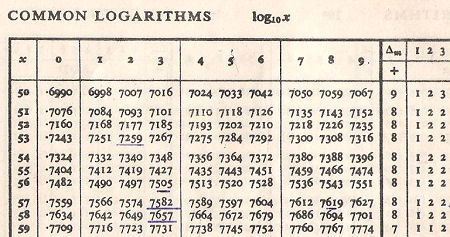A table of common logarithms. [Image source]

The slide rule is a device that also relied on ratios of numbers to simplify tedious calculations.

## Logarithms to Base e

In most scientific and computing applications, logarithms to base e are used. (e = 2.718281828459..., an important and common mathematical constant.)

It is so common in fact, that "log" is assumed to mean "log base e" in many scientific situations (the opposite of what it means on your calculator).

In many computing languages, it is also assumed "log" means natural logarithm "log base e"), and this only adds to the confusion for engineering students.

## Why Do We Still Learn Logarithms?

Even though we don't use logs any more for multiplication and division, the logarithm function (and its related exponential function) are still very commonly used in science and engineering. Logarithms are also important in financial and population studies.

You an see more on logarithms here: Exponential and Logarithmic Functions

## Let's Fix Logarithm Notation

Many students, like Yousuf, get unnecessarily confused about logarithms because of the poor notation used.

Natural logarithms: I say we should drop "ln" notation altogether and use "loge" only, in both text books and on calculators.

Common logarithms: We should only use "log10" notation for common logarithms on calculators and text books.

Even in computer programming we could use "loge" (no subscript needed) for natural log, and maybe "logc" for common logarithms.

Do you agree with my suggestion?

### 24 Comments on “Logarithms - a visual introduction”

1. Joe says:

I agree, but I think the problem is bigger than that.

The convention of writing powers as superscripts is where we went wrong in the first place, but that's for another post.

Even the functional notation "exp(x)" I have found to be unnecessarily confusing even for students familiar with "sin(x)".

Maybe what we need is a special symbol alongside +, ?, × (or ? or *), and ÷ (or : or / or the fraction bar interposed between numerator and denominator) and dispense with superscripts and "?" with its overbar. The "^" symbol is already sanctioned by common use owing to the influence of the keyboard.

At the same time, the operation ^ has two inverses. How about "{" and "}", so that 2 ^ 3 = 8 iff 2 = 8 } 3 and 3 = 8 { 2, just as 1 + 2 = 3 iff 1 = 3 - 2 and 2 * 3 = 6 iff 2 = 6 / 3?

Hence, while "loge(x)" and "logc(x)" are notations that build naturally on documentary precedent, why not eliminate functional notation altogether and symbolize loga(b) as a { b?

2. Murray says:

Thanks for the suggestion, Joe. Certainly food for thought there.

I agree ^ is preferable to superscript a lot of the time, but when the complexity increases in certain formulas, it is harder to read.

In an earlier post I suggested another alternative to function notation which you may find interesting: Towards More Meaningful Math Notation. It partially addresses the "2 inverses" problem you mentioned.

3. Sue VanHattum says:

Wolfram Alpha, a force to be reckoned with, uses what you've called the engineering notation. log (100) gives 4.6, because it's log base e.

4. Sue VanHattum says:

Oops! meant to say...

I love the history, and the exponential graphs. I've bookmarked this post to show my students.

5. Placido says:

I think it's a great idea to change log notation. I always get confused, especially withthe base.

6. VE says:

It is always stated that logarithms were developed in order to make the multiplication and division of large numbers easier. I think, the initial hurdle students have, including myself when I learned logs, is that they have to initially go through understanding the notation and the laws of logs in order to grasp how this is possible.

But I always wondered how logs were used initially, by Napier and Briggs. Were their logarithms the same as how we use them today? This post was the impetus to renew the search for those answers. Thanks to today’s technology and the World Wide Web, I was able to come by those solutions.

Following are links to great descriptions on the history of logarithms has well the use of log tables, and the slide rule. Hope your readers enjoy.

History of Logs, Napier’s original logs, and How to Use a Log Table
[no longer available]

Someone fondly remembers the Slide Rules and other links to understanding its use.
http://goodmorningyesterday.blogspot.com/2007/06/slide-rule.html

7. Murray says:

Thanks for the references, VE.

I alluded to the strangeness of Napier's original base - but it was certainly better than nothing!

8. Justin Moretti says:

It would be good to see the return of the slide rule, I think, particularly in High School physics labs. In pure maths it may be acceptable to run answers out to however many S.F. you care to name, but in physics (especially in practical lab work) it would bring home to the students the limitations of accuracy in the measurements they're making and hence the accuracy of their results.

9. Murray says:

Yes, good point Justin. Students only really get a sense of significant digits from measuring things, not from chopping or rounding numbers.

10. Travels in a Mathematical World says:

Carnival of Mathematics #67...

On the subject of poorly designed notation, don't get Murray Bourne of squareCircleZ started on the notation for natural logarithm! In Logarithms ? a visual introduction, he motivates logarithms from a historical perspective, and uses an example to sh...

11. Garrett Mackelprang says:

I am a high school drop out, and from this perspective saw logarithms as strange and mysterious. After siting down for about an hour and playing around with Microsoft Mathematics, I finally came to understand what they are all about. The biggest hurdle is to see things as x & y axis points. One of the biggest things that helped me was understanding that PLOT of log10 is y=10^x this simplified becomes (x root of(y))=10 OR (y^(1/x))=10 WHICH IS MORE POWERFULL THAN log10(x). THIS is what all that mysterious "log(x)" stuff is but more powerful in the sense that you can get the "anitlog" (y value from x value) and the log (x value from the y value) very quickly with one formula, just put in the x or y value and get out the corresponding y or x value (using a calculator of course). Thus all the "strange" rules for logs are seen as the same of those of a arbitrary number to some arbitrary root or fractional power. It would be nice if you would piece this into your already excellent article above, I believe if I saw what I discovered on my own before spending all the time trying to figure it out, it would have speed up my understanding of logs.

12. Murray says:

13. Garrett Mackelprang says:

I gave it some more thought and came to the conclusion that this idea is quite use full for finding the base of an unknown log and also for any arbitrary log that you need for a specific circumstance. My circumstances were in the understanding of an exponential rise (1-(e^-1)) or fall (e^-1) curves. (Within limits y=1max & x=5max, this region is the only section of the two plots used in my personal study)

This in a nut shell:

Plot of y=b^x is the arbitrary curve being studied, where b is any arbitrary base (other than zero or +-1), (x,0) is a linear length along the x axis and (0,y) is a nonlinear length along the y axis.

Therefore we get for our arbitrary plotted curve being studied:

y=b^x
b=(x root of(y)
x=logb(y)

From here you can find the base b of an unknown log (if a point y and its corresponding point x are known) or any point for y if x and b are known with the same being true for finding x.

This used in a "real life" example for the decay (exponential fall) of a capacitors stored energy into a resistance:

b=(e^-1)=(x root of(y) (the base used is that of exponential fall)
y=(e^-x) (y=magnitude, in percent)
x=loge^-1(y)=(-1)loge(y) (x=time constant "Tau"=(rC))

(where rC corresponds to seconds in time)

Plotting limits y=1max & x=5max (when practically considered, other wise x=infinity or some arbitrary number usually greater than 5)

To find the 50% magnitude or half the voltage of the capacitor:
y=0.5 (the 50% mark or half voltage)
x=loge(2)=(-1)loge(0.5)

This math stuff interpreted comes out to be 0.69314 * rC equals the point in time that the voltage is one half of its prior maximum.

Boring I know but this helped me out a bunch so I thought I would share this with a real life use example as well.

14. Murray says:

Not boring, Garrett! Thanks for sharing.

15. abhishek says:

Calculation with the help of Commonlog base 10 in logbook give accurecy of only upto the 4 decimal places.
Q. Can u suggest any logtable u have! PDF copy as authentic copy or any other which gives result with accuracy give upto 9 decimal places.

16. Murray says:

@abhishek: I couldn't find a 9-decimal place accurate log table, so I wrote one.

Go to Interactive Logarithm Table. You can choose from a few different bases, and up to 9 decimal place accuracy.

If you really need a PDF, you can highlight the portion you want, save it into Word (say), and then convert to PDF.

17. David Geltzer says:

Hi,

Please explain how I calculate the growth of a nautilus shell among other such natural phenomenon. I am especially interested in knowing how to calculate the increase of the radius every 360 degrees around. If you have a formula that would be great.

Thank you,

David G

18. Murray says:

@David: In the first example on this page, https://www.intmath.com/plane-analytic-geometry/equiangular-spiral.php, the ratio of one radius to the next is: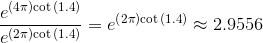You can see in the spiral image that the largest radius shown is about 37 units, and the next one to the left (on the horizontal axis) is around 12 (which is about 1/3 of the largest one) and the next smaller one is about 4 units (once again, 1/3 of the one before.

Hope it helps.

19. Kurt says:

Are there any standard for what values should be used for the minor ticks/grids in between the main ones of a base 10 log chart axis?

20. Murray says:

@Kurt: This page shows the values on the minor grids for log-log graphs: https://www.intmath.com/exponential-logarithmic-functions/7-graphs-log-semilog.php

It's the 8 (normally evenly-spaced) values between the surrounding major ticks. For example, the minor ticks between 10 and 100 will be 20, 30, 40, 50, ... 80, 90.

21. Kurt says:

The reason I was asking, is that some software components that I'm evaluating are not using the 8 evenly spaced values between the major ticks. Is this "wrong", or is there no written standard for how this should be done.

22. Murray says:

@Kurt: Actually, the minor ticks are evenly spaced on a linear grid, but unevenly spaced when using log axes.

It doesn't hurt to miss out the minor ticks. The main thing is the major ticks need to be labelled clearly.

23. abe says:

Thanks so much for the clear explanation of logarithms! This is the best explanation I have ever read! It really helped me grasp the concept!

24. Daniel Lonergan says:

A log is used to make large numbers small to fit on a paper page. They are simply the power (exponent) on the base in question (usually 10 as in ) that will provide the large number in a less lengthy form thereby promulgating a snap shot visualization of graphical simplicity.

### Comment Preview

HTML: You can use simple tags like <b>, <a href="...">, etc.

To enter math, you can can either:

1. Use simple calculator-like input in the following format (surround your math in backticks, or qq on tablet or phone):
a^2 = sqrt(b^2 + c^2)
(See more on ASCIIMath syntax); or
2. Use simple LaTeX in the following format. Surround your math with $$ and $$.
$$\int g dx = \sqrt{\frac{a}{b}}$$
(This is standard simple LaTeX.)

NOTE: You can mix both types of math entry in your comment.